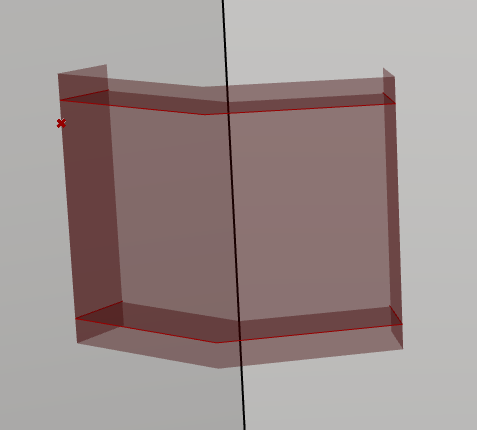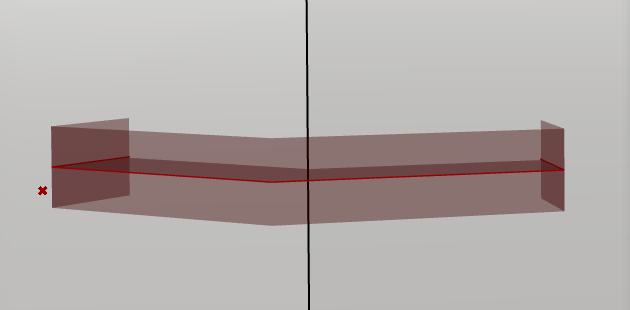# Mesh Extrusion not working as expected

I’m using the Rhino.Geometry.Mesh.CreateFromCurveExtrusion Method, like this:

``````  private void RunScript(DataTree<Curve> crv_Profile, DataTree<Polyline> poly_Profile1, DataTree<Polyline> poly_Profile2, Vector3d extr_Vec, DataTree<Point3d> in_BboxPts, ref object A)
{
List<Mesh> output = new List<Mesh>();
var mesh_Params = Rhino.Geometry.MeshingParameters.QualityRenderMesh;

for (int i = 0; i < crv_Profile.BranchCount; i++){
for (int j = 0; j < crv_Profile.Branch(i).Count; j++){
Rhino.Geometry.BoundingBox box = new Rhino.Geometry.BoundingBox(in_BboxPts.Branch(i, j), in_BboxPts.Branch(i, j));
Mesh out_Mesh = Rhino.Geometry.Mesh.CreateFromCurveExtrusion(crv_Profile.Branch(i)[j], extr_Vec, mesh_Params, box);
Mesh mesh_Pline1 = Rhino.Geometry.Mesh.CreateFromClosedPolyline(poly_Profile1.Branch(i)[j]);
Mesh mesh_Pline2 = Rhino.Geometry.Mesh.CreateFromClosedPolyline(poly_Profile2.Branch(i)[j]);
out_Mesh.Append(mesh_Pline1);
out_Mesh.Append(mesh_Pline2);
}
}
A = output;
``````

The result has the mesh going past the expected top and bottom. If I change the extrusion vector length to very small 0.01, there are still two parts sticking out of the top and bottom. Does anyone know what is going on here? Here are some pictures to describe the problem:Amplitude is 8.5Amplitude 0.01

I would really like to know what is going on here. The bounding box is extending beyond what is expected by exactly 1.0 units above and below.

You’re right. It behaves exactly the same for me. Changing the direction vector also doesn’t do anything. Using a bounding box to scale the extrusion also seems weird to me.

Anyhow, if you only want to create something like a mesh loft between two or more polylines with optional end caps, here’s a Python example of mine:

``````"""Create a lofted mesh through a set of section polylines."""

__author__ = "diff-arch (https://diff-arch.xyz)"
__version__ = "1.2.1 (2021-10-16)"

import Rhino.Geometry as rg
import scriptcontext as sc
import Grasshopper as gh
import math

class MeshLoft:
"""Creates a lofted mesh through a set of section polylines.

Attributes:
polylines (list): Valid, closed or open polylines to loft
close_loft (bool): Optional, if True the loft will be closed
cap_loft (bool): Optional, if True the loft will be capped

To use:
>>> ml = MeshLoft(polylines)
>>> loft = ml.mesh
"""
def __init__(self, polylines, close_loft=None, cap_loft=None):
"""Inits this mesh loft."""
self.polylines = polylines
self.close_loft = close_loft
self.cap_loft = cap_loft
self.vertices = self.get_vertices()
self.faces = self.construct_faces()
self.mesh = self.construct_mesh()

def get_vertices(self):
"""Gets the vertices for each polyline in self.polylines.

Returns:
The new nested list of vertices per polyline.
"""
pline_vertices = []
for pline in self.polylines:
vertices = []
for i in xrange(len(pline)):
vertices.append(pline[i])
pline_vertices.append(vertices)
return pline_vertices

def index_vertices(self):
"""Indexes the vertices of each polyline with integer numbers,
that are necessary to later assign the vertices to mesh faces.

Returns:
The nested list of vertex indices (e.g. [[0,1,2], [3,4,5], ..]).
"""
vtx_indices = []
for i in xrange(len(self.vertices)):
indices = []
for j in xrange(len(self.vertices[i])):
indices.append(i * len(self.vertices[i]) + j)
vtx_indices.append(indices)
return vtx_indices

def construct_faces(self):

Returns:
The nested list of face tuples (e.g. [(0,1,7,6), ...]).
"""
vtx_indices = self.index_vertices()
faces = []
for i in xrange(len(vtx_indices)):
if i < len(vtx_indices) - 1:
# Loop the polyline vertex indices up to the last curve
for j in xrange(len(vtx_indices[i])-1): # vertices
v1 = j + (i * len(vtx_indices[i]))
v2 = j + (i * len(vtx_indices[i])) + 1
v3 = j + ((i + 1) * len(vtx_indices[i])) + 1
v4 = j + ((i + 1) * len(vtx_indices[i]))
faces.append((v1, v2, v3, v4))
else:
# Loop the polyline vertex indices of the last curve
if self.close_loft:
for j in xrange(len(vtx_indices[i])-1): # vertices
v1 = j + (i * len(vtx_indices[i]))
v2 = j + (i * len(vtx_indices[i])) + 1
v3 = j + 1
v4 = j
faces.append((v1, v2, v3, v4))
return faces

def construct_mesh(self, angle_tolerance=-1.0):
"""Constructs a lofted mesh from self.vertices and self.faces
and adds caps to it, if necessary.

Args:
angle_tol (float): An optional angle tolerance for vertex welding

Returns:
The new mesh loft.
"""
if angle_tolerance == None or angle_tolerance <= 0:

mesh = rg.Mesh()

# Add the mesh loft vertices
for pline_vertices in self.vertices:
for vertex in pline_vertices:
# Add the mesh loft faces
for face in self.faces:
v0, v1, v2, v3 = face
if self.cap_loft != None and self.cap_loft > 0:
end_curves = [self.polylines, self.polylines[-1]]
if self.cap_loft == 1: # flat cap
mesh_caps = [self.construct_flat_cap(c) for c in end_curves]
cap_fails = 0
for cap in mesh_caps:
if cap != None:
mesh.Append(cap)
mesh.Weld(angle_tolerance)
mesh.Vertices.CombineIdentical(False, False)
else:
cap_fails += 1
if cap_fails == len(end_curves):
err = "Both caps failed."
gh.Kernel.GH_RuntimeMessageLevel.Error, err)
if cap_fails == len(end_curves) - 1:
err = "One cap failed."
gh.Kernel.GH_RuntimeMessageLevel.Error, err)
# Compute the normals and compact the mesh
mesh.Normals.ComputeNormals()
mesh.Compact()
return mesh

def construct_flat_cap(self, edge, tolerance=None):
"""Constructs a flat mesh cap for a closed, naked mesh edge.

Edge polylines with a count of 4 or 5 vertices produce single,
triangular or quad faces. A count of more than 5 edge vertices
results in a triangulated mesh. If these edge vertices share a
center vertex contained inside the naked edge, the face count
equals the number of edge segments, and the faces are radially
oriented around this center vertex. If the center vertex lies
outside the closed mesh edge, the triangulated mesh is produced
from the edge polyline itself.

Args:
edge (Rhino.Geometry.Polyline): A polyline representing a
closed, naked mesh edge
tolerance (float): An optional tolerance for planar projection

Returns:
The new mesh cap on success, or None.
"""
if tolerance == None or tolerance <= 0:
tolerance = sc.doc.ModelAbsoluteTolerance

mesh_cap = rg.Mesh()
# Test for planarity of the mesh edge polyline
pcurve = rg.PolylineCurve(edge)
is_planar, plane = pcurve.TryGetPlane()
if not is_planar:
# Construct a planar test edge from the non-planar one
rc, plane = rg.Plane.FitPlaneToPoints(edge)
proj_pts = [plane.ClosestPoint(pt) for pt in edge]
pcurve = rg.PolylineCurve(proj_pts)
# Find the center vertex of the naked mesh edge
center_vtx = edge.CenterPoint()
# Test for center vertex mesh edge containment
rc = pcurve.Contains(center_vtx, plane, tolerance)
if rc == rg.PointContainment.Outside \
or rc == rg.PointContainment.Coincident:
# Mesh cap corresponds to a triangulated polyline
mesh_cap = rg.Mesh.CreateFromClosedPolyline(edge)
if rc == rg.PointContainment.Inside:
if edge.Count - 1 == 3:
# Mesh cap is composed of a single tri face
for i in xrange(edge.Count - 1):
if edge.Count - 1 == 4:
# Mesh cap is composed of a single quad face
for i in xrange(edge.Count - 1):
if edge.Count > 5:
fragments = shatter_at_kinks(edge)

if all(s.SegmentCount == 2 for s in fragments):
discs = get_discontinuities(edge)
closest_disc_idx, closest_dist_sq = find_closest_point(edge.First, discs)

if closest_dist_sq > sc.doc.ModelAbsoluteTolerance:
# Adjust the edge start vertex to a discontinuity one
closest_vtx_idx, _ = closest_point(discs[closest_disc_idx], edge)

for i in xrange(edge.Count):
for i in xrange(0, edge.Count - 1, 2):
v0 = i
v1 = i + 1
v2 = edge.Count
v3 = edge.Count-2 if i == 0 else i - 1

else:
# Mesh cap is radially triangulated around the center vertex
for i in xrange(edge.Count):
for i in xrange(edge.Count - 1):
v0 = i
v1 = i + 1
v2 = edge.Count

if rc == rg.PointContainment.Unset:
err = "Open polylines can't produce capped mesh lofts."
gh.Kernel.GH_RuntimeMessageLevel.Warning, err)
return
# Compute the normals and compact the mesh
mesh_cap.Normals.ComputeNormals()
mesh_cap.Compact()
return mesh_cap

######################## UTILITY FUNCTIONS #########################

def align_polylines(polylines, guide_idx=None):
"""Aligns closed polylines so that their start/end vertices fall inline.
If the optional guide index is not defined, the polylines align to
the start/end vertex of the first polyline.

Args:
polylines (list): A list of closed polylines
guide_idx (int): An optional guide index for the polylines to align to

Returns:
The list of aligned polylines on success, or the unaltered polylines.
"""
if any([True for p in polylines if not p.IsClosed]):
err = "Open polylines can't be aligned."
gh.Kernel.GH_RuntimeMessageLevel.Warning, err)
return polylines

if guide_idx == None or guide_idx < 0:
guide_idx = 0
elif guide_idx > len(polylines):
guide_idx = 0

aligned_polylines = [polylines[guide_idx]]
for i in range(1, len(polylines)):
# Get the guide vertex of the previous polyline
guide_vtx = polylines[i-1][guide_idx]
# Get the closest index of a vertex of the current polyline
closest_idx = polylines[i].ClosestIndex(guide_vtx) # -1 on error
if closest_idx < 0:
return
# Get a list of indices of the current polyline
indices = range(len(polylines[i])-1)
# Restructure the polyline indices to align them
align_indices = indices[closest_idx:] + indices[:closest_idx]
align_vertices = [polylines[i][j] for j in align_indices]
align_vertices.append(align_vertices)
aligned_polylines.append(rg.Polyline(align_vertices))
# Update the guide index
guide_idx = closest_idx
return aligned_polylines

"""Shatters a polyline into sections at sharp kinks.

Args:
pline (Rhino.Geometry.Polyline): A polyline to shatter
for a break to happen, by default the document angle tolerence

Returns:
A list of polyline segments.
"""
segments = pline.GetSegments()
fragments = [rg.Polyline()]
for i in xrange(pline.SegmentCount):
current_seg = segments[i]
next_seg = segments if i == pline.SegmentCount-1 else segments[i+1]
angle = rg.Vector3d.VectorAngle(
current_seg.UnitTangent,
next_seg.UnitTangent
)
if angle < tol:
if i == pline.SegmentCount-1:
if pline.IsClosed:
fragments.Insert(0, current_seg.From)
fragments.pop()
else:
continue
if i < pline.SegmentCount - 1:
fragments.append(rg.Polyline())
return fragments

"""Returns all discontinuities along a polyline."""
fragments = shatter_at_kinks(pline, tol)
vertices = []
for frag in fragments:
vertices.append(frag.First)
if not pline.IsClosed:
vertices.append(fragments[-1].Last)
return vertices

def find_closest_point(test_pt, points):
"""Finds the closest point to a test point in a list of points.

Args:
test_pt (Rhino.Geometry.Point3d): Point to search from
points (list): Collection of points to search

Returns:
The index of the closest point  and the squared distance 
between it and the test point.
"""
min_dist_sq = float("inf")
closest_idx = None
for i, pt in enumerate(points):
dd = test_pt.DistanceToSquared(pt)
if dd < min_dist_sq:
min_dist_sq = dd
closest_idx = i
return closest_idx, min_dist_sq

"""Adjusts the seam or start point of a closed polyline.

Args:
pline (Rhino.Geometry.Polyline): A polyline to adjust
index (int): Index of a polyline vertex to move the seam to

Returns:
"""
if not pline.IsClosed():
return pline
new_pline = rg.Polyline()
for i in xrange(index, pline.Count, 1):
for i in xrange(1, index+1):
return new_pline
``````

The vertices of the input polylines define the number of mesh divisions in u-direction and their number the divisions in v-direction. It’s thus easy to control the mesh subdivisions.

Should be pretty straightforward to translate to C#.

Thanks, this is helpful. I am still baffled by that extra extrusion, but I will just use this script in the meantime. I imagine it is pretty fast.

Hi Devin,

Have you sorted this out? I am having the same issue - one unit above and below. Driving me nuts…

I was never able to get the mesh extrusion working how I thought it should and since the measurements of the extrusion were not exactly the amounts I thought when trying to work around this quirk, I had to just manually make the mesh extrusion by building out all of the faces and creating the mesh.

I see. I ended up deducting and adding up one unit from top and bottom hoping this to be consistent. This must be a bug, isn’t it?

Just so you know, from my tests, it’s not exactly 1 unit. But I do think this is a bug and I wish someone at McNeel that knows more about this could chime in. I would really love to be able to use this function more frequently. It can generate some really nice, simple mesh extrusions.

Thanks for submitting it separately to get their attention (hopefully)

Yeah, hopefully, they can fix it soon.